Click on the magic wand to donate now!

# There's Still Time to Participate!

## Challenge Ends at Midnight on Tuesday, January 8

It's magical to watch this Annual Appeal Challenge grow. Five Northside donors have offered to match your donation, of any amount, up to a total of \$30,000.

The good news is that we are almost to our match goal. Make a donation today to help us meet our goal and turn \$30,000 into \$60,000. Magic!

However, we are still \$37,000 away from our goal for the overall Annual Appeal. We need to close this gap. Can we count on you?

All of our children benefit from the services Friends of Northside helps to provide. Every dollar helps!

Final Challenge results will be announced on January 13.## Thank you to everyone who has participated so far!

Remember, you can make a donation to the Annual Appeal at any time.

 table div table+table+table+table+table+table+table div table{width:100%;padding:0}table div table+table+table+table+table+table+table div table img{width:96.23%;padding:0;float:none}table div table+table+table+table+table+table+table div table td{width:100%;padding:0 1.88% 18px}/* styles */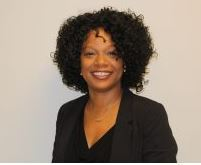# From Principal Mest

Dear Northside Families,

Welcome back and Happy New Year!

I hope that you had a wonderful winter break with time to relax, spend time with loved ones, and to re-charge for this new year.

As we return to school tomorrow, we have a great deal in store for us this month: a dance showcase, Homecoming/Spirit Week, a whole school colloquium day, finals week, and so much more. And because I suspect that it will only take us a few days to get back into our collective NCP rhythm, I believe that the start of a new year is a perfect time for our community re-center ourselves around the mission, values, and beliefs that drive the work that we do.

The Mission of Northside College Preparatory High School is:
To create a responsive environment that challenges all to embrace the joy of discovery, nurtures learners as they strive to fulfill their individual and collective potential, and empowers students to pursue their roles in the local and global communities.

We value ENGAGEMENT and believe...

▪ learners thrive most when they value and relish the learning of the moment, in and for itself, not simply as a means to a later goal.
▪ every person is a learner and a teacher who constructs meaning and understanding.
▪ reflection on both failure and success is necessary for growth.
 ▪ learners thrive most when they value and relish the learning of the moment, in and for itself, not simply as a means to a later goal.
 ▪ every person is a learner and a teacher who constructs meaning and understanding.
 ▪ reflection on both failure and success is necessary for growth.

We value ETHICS and believe...

▪ ethical behavior underlies each individual’s endeavors, and self-respect is built upon achievement and integrity.
▪ individuals enrich the democratic values and practices of the society in which they live.
▪ all must accept the challenge to share their gifts with others for the betterment of the world in which they live.
 ▪ ethical behavior underlies each individual’s endeavors, and self-respect is built upon achievement and integrity.
 ▪ individuals enrich the democratic values and practices of the society in which they live.
 ▪ all must accept the challenge to share their gifts with others for the betterment of the world in which they live.

We value CURIOSITY and believe...

▪ creative exploration and expression foster curiosity and deeper understanding.
▪ student inquiry and interests influence educational experiences in and out of the classroom.
▪ it is important to recognize experiences beyond the school walls and celebrate them as powerful drivers of learning.
 ▪ creative exploration and expression foster curiosity and deeper understanding.
 ▪ student inquiry and interests influence educational experiences in and out of the classroom.
 ▪ it is important to recognize experiences beyond the school walls and celebrate them as powerful drivers of learning.

We value COLLABORATION and believe...

▪ people grow and learn through challenging themselves and collaborating with others.
▪ people share credit and take responsibility for their work.
▪ students, parents, teachers, administrators, and community leaders create and sustain a nurturing learning environment.
 ▪ people grow and learn through challenging themselves and collaborating with others.
 ▪ people share credit and take responsibility for their work.
 ▪ students, parents, teachers, administrators, and community leaders create and sustain a nurturing learning environment.

We value EMPATHY and believe...

▪ a meaningful education balances academic, social, and emotional needs and experiences.
▪ we all must recognize, understand, and acknowledge different perspectives and experiences and value how they enrich our community.
▪ in speech and action we look beyond ourselves and consider our impact on others.
 ▪ a meaningful education balances academic, social, and emotional needs and experiences.
 ▪ we all must recognize, understand, and acknowledge different perspectives and experiences and value how they enrich our community.
 ▪ in speech and action we look beyond ourselves and consider our impact on others.

Thank you for all that you do to support your student and the work of the NCP community. I look forward to how we will learn and grow together in 2019.

Warm Regards,

Kelly Mest

 table div table+table+table+table+table+table+table+table+table div table{width:100%;padding:0}table div table+table+table+table+table+table+table+table+table div table img{width:96.23%;padding:0;float:none}table div table+table+table+table+table+table+table+table+table div table td{width:100%;padding:0 1.88% 18px}/* styles */# Thank You, Northside Families

A big thank you to all the families who donated food, drinks, supplies, flowers, thank you notes, gift cards and time for the Faculty and Staff Appreciation Holiday Buffet. It was a wonderful day at Northside, enjoyed and very much appreciated by all.

We collected over 100 gift cards which meant that each and every one of our faculty and staff received one. Wow!

Many thanks,

Nancy Liljedahl, Christine Lee, and Brian Knight

 table div table+table+table+table+table+table+table+table+table+table+table div table{width:100%;padding:0}table div table+table+table+table+table+table+table+table+table+table+table div table img{width:96.23%;padding:0;float:none}table div table+table+table+table+table+table+table+table+table+table+table div table td{width:100%;padding:0 1.88% 18px}/* styles */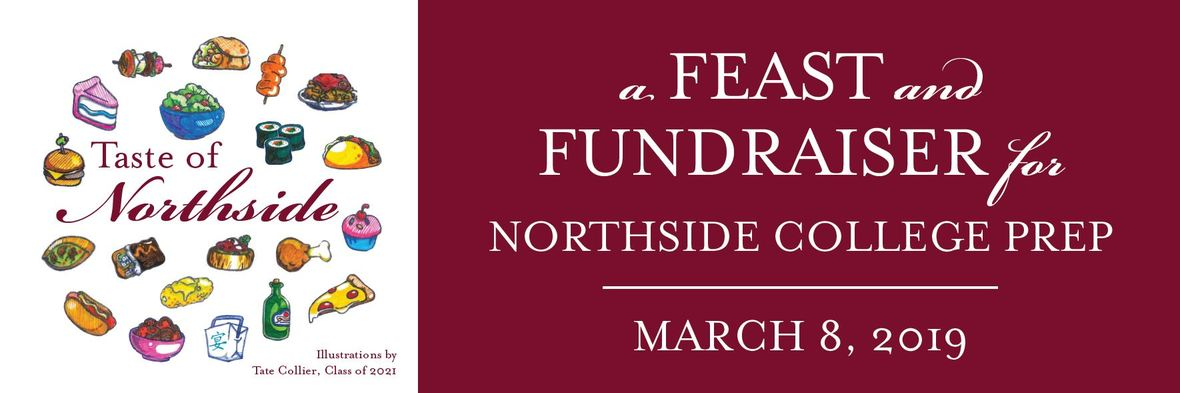# Getting Ready for the 2019 Spring Benefit## Auction Items Needed

The solicitation committee needs your help to secure more items for the live and silent auctions – sports tickets, restaurant gift cards, travel and hospitality vouchers, tutoring, unique experiences, hosted parent parties, etc. Please email Kristen Werries Collier, kristenwerriescollier@gmail.com, to donate, get more information, or pass on connections to pursue. Click HERE for the donation form.Corporate Sponsorship contributions will be used to underwrite the cost of the 2019 Spring Benefit and enable Friends of Northside to provide more of its financial resources for programs and services for the school not covered by the CPS budget. For sponsorship opportunities and in-kind donations, please contact Matt Schechter, matthew.d.schechter@gmail.com. Click HERE for the sponsorship form.We are putting together a slideshow of our students participating in the many activities that make Northside special. If you have pictures you'd like to be considered for inclusion in the presentation, please forward them to Debra Swan at debra@theswans.net.

## Food & Beverages Needed

Have a connection to a local restaurant or beverage vendor? We need items to add to our dinner buffet, as well as wine, champagne, and soda. Contact Debra Swan, debra@theswans.net, if you can help.

## Build a Social Experience for NCP

A fun aspect of our annual Benefit fundraising effort each year is the Parent Parties! Northside families offer to host a party for other Northside parents and families. The host pays for the party, guests pay to attend and the revenue generated from tickets is all donated to Northside. These parties raise \$500-\$2500 each and are a great opportunity to meet and mingle with other Northside parents. A few ideas are Moms & Margaritas, Poker Night, Wine Tasting, Backyard BBQ, Theme Dinner Party, and more.

Please consider hosting a party like one of those suggested, come up with your own idea or we can help you put something together. Reach out to Nancy Liljedahl at n.liljedahl@comcast.net if you are interested in hosting or want to brainstorm. Thanks for your support!

 table div table+table+table+table+table+table+table+table+table+table+table+table+table+table+table+table+table+table+table div table{width:100%;padding:0}table div table+table+table+table+table+table+table+table+table+table+table+table+table+table+table+table+table+table+table div table img{width:96.23%;padding:0;float:none}table div table+table+table+table+table+table+table+table+table+table+table+table+table+table+table+table+table+table+table div table td{width:100%;padding:0 1.88% 18px}/* styles */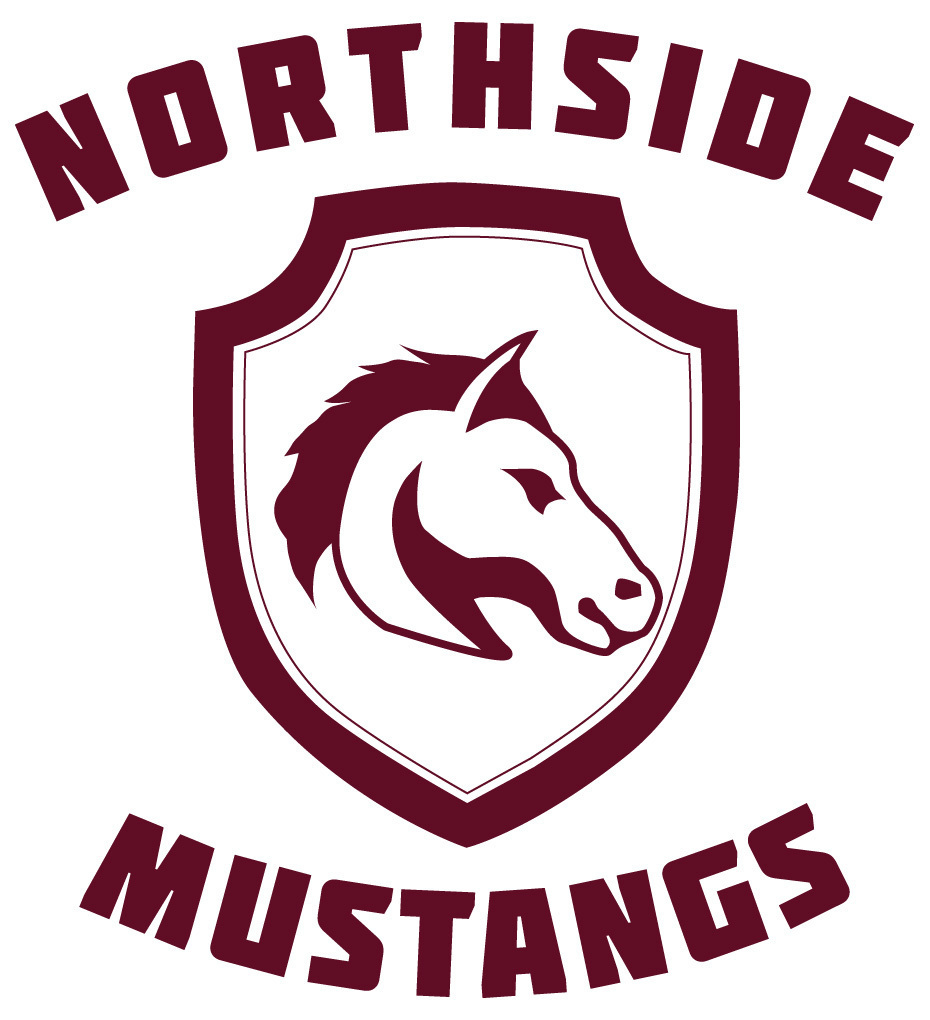# NCP Athletic Boosters Meeting

Thursday, January 10
7:00 PM
Faculty Lounge

 table div table+table+table+table+table+table+table+table+table+table+table+table+table+table+table+table+table+table+table+table+table div table{width:100%;padding:0}table div table+table+table+table+table+table+table+table+table+table+table+table+table+table+table+table+table+table+table+table+table div table img{width:96.23%;padding:0;float:none}table div table+table+table+table+table+table+table+table+table+table+table+table+table+table+table+table+table+table+table+table+table div table td{width:100%;padding:0 1.88% 18px}/* styles */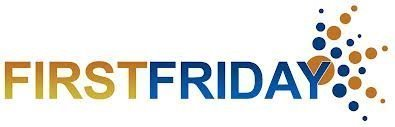/* styles */ Friday, January 11 8:00 - 10:00 am Panera Bread, 6059 N. Lincoln Ave Join fellow NCP parents at Friends of Northside's monthly coffee gathering. Stay for as long as your schedule allows.
 table div table+table+table+table+table+table+table+table+table+table+table+table+table+table+table+table+table+table+table+table+table+table+table+table div table{width:100%;padding:0}table div table+table+table+table+table+table+table+table+table+table+table+table+table+table+table+table+table+table+table+table+table+table+table+table div table img{width:96.23%;padding:0;float:none}table div table+table+table+table+table+table+table+table+table+table+table+table+table+table+table+table+table+table+table+table+table+table+table+table div table td{width:100%;padding:0 1.88% 18px}/* styles */# Dance Showcase

Friday, January 11
7:00 pm
Auditorium

 table div table+table+table+table+table+table+table+table+table+table+table+table+table+table+table+table+table+table+table+table+table+table+table+table+table+table div table{width:100%;padding:0}table div table+table+table+table+table+table+table+table+table+table+table+table+table+table+table+table+table+table+table+table+table+table+table+table+table+table div table img{width:96.23%;padding:0;float:none}table div table+table+table+table+table+table+table+table+table+table+table+table+table+table+table+table+table+table+table+table+table+table+table+table+table+table div table td{width:100%;padding:0 1.88% 18px}/* styles */# This Week in Sports

Come to a game and support our student athletes!

▪ Thursday, January 10 at 5:00 pm (V) and 6:30 pm (SO) vs. Crane at Crane
 ▪ Thursday, January 10 at 5:00 pm (V) and 6:30 pm (SO) vs. Crane at Crane

▪ Wednesday, January 9 at 5:00 pm (V) and 6:30 pm (SO) vs. Alcott at Alcott
▪ Friday, January 11 at 5:00 pm (V) and 6:30 pm (SO) vs. Mather HS at Mather HS
 ▪ Wednesday, January 9 at 5:00 pm (V) and 6:30 pm (SO) vs. Alcott at Alcott
 ▪ Friday, January 11 at 5:00 pm (V) and 6:30 pm (SO) vs. Mather HS at Mather HS

## Women’s Bowling

▪ Monday, January 7 at 4:15 pm vs. Lincoln Park HS at Home (Waveland Bowl)
▪ Saturday, January 12 at 10:00 am vs. TBA at Home (Waveland Bowl)
 ▪ Monday, January 7 at 4:15 pm vs. Lincoln Park HS at Home (Waveland Bowl)
 ▪ Saturday, January 12 at 10:00 am vs. TBA at Home (Waveland Bowl)

## Men’s Swimming and Diving

Practices began on Monday, November 19. Email Coach Ceja for more information.

## Men’s & Women’s Indoor Track & Field

Indoor season will begin on Monday, January 14. Contact Director of Athletics Henderson for details.

## Wrestling

The wrestling team will co-op with Von Steuben High School this year. Contact Director of Athletics Henderson for details.

 table div table+table+table+table+table+table+table+table+table+table+table+table+table+table+table+table+table+table+table+table+table+table+table+table+table+table+table+table+table div table{width:100%;padding:0}table div table+table+table+table+table+table+table+table+table+table+table+table+table+table+table+table+table+table+table+table+table+table+table+table+table+table+table+table+table div table img{width:96.23%;padding:0;float:none}table div table+table+table+table+table+table+table+table+table+table+table+table+table+table+table+table+table+table+table+table+table+table+table+table+table+table+table+table+table div table td{width:100%;padding:0 1.88% 18px}/* styles */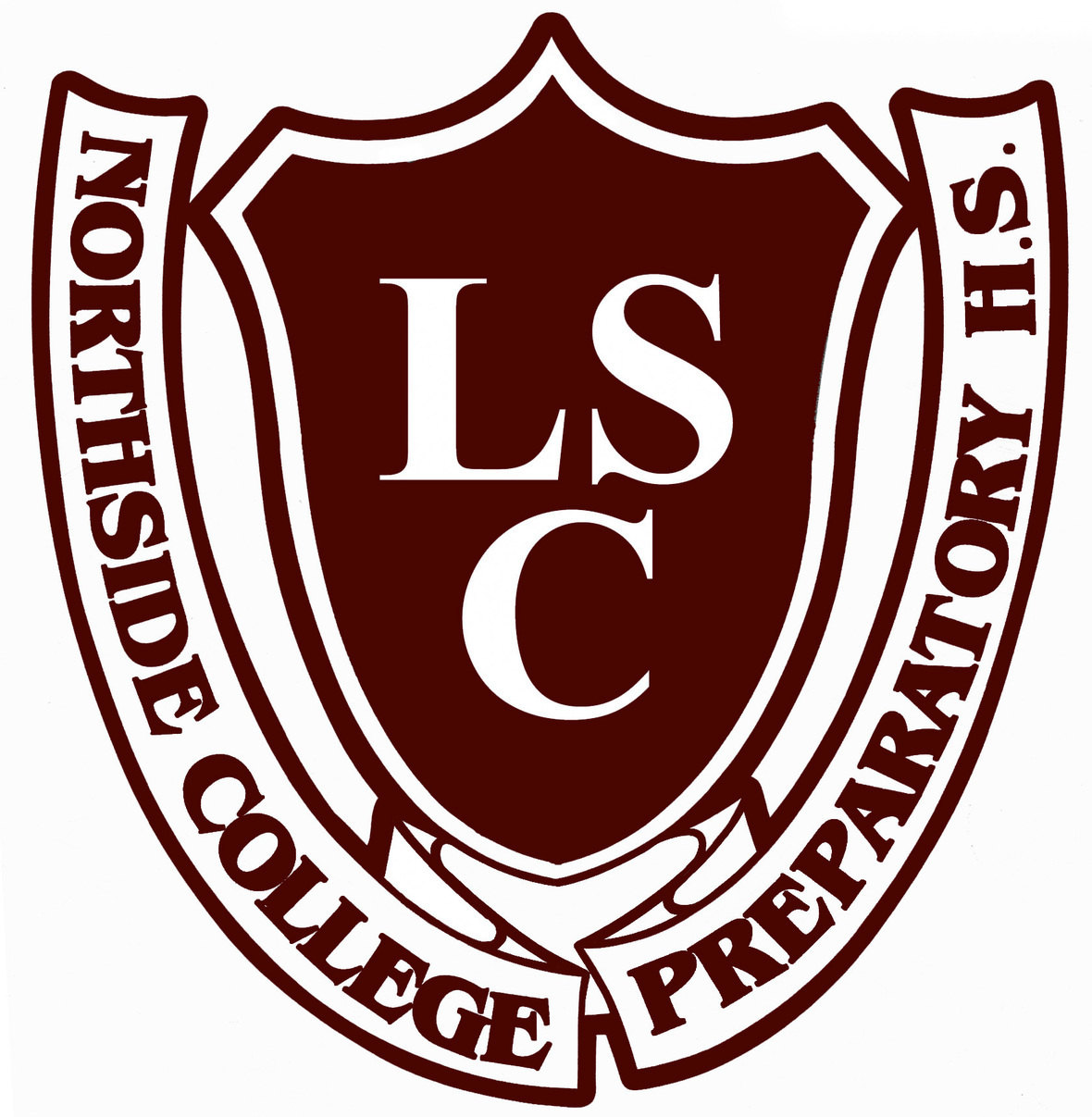# LSC Meeting

Tuesday, January 15
6:30 pm
Faculty Lounge

 table div table+table+table+table+table+table+table+table+table+table+table+table+table+table+table+table+table+table+table+table+table+table+table+table+table+table+table+table+table+table+table div table{width:100%;padding:0}table div table+table+table+table+table+table+table+table+table+table+table+table+table+table+table+table+table+table+table+table+table+table+table+table+table+table+table+table+table+table+table div table img{width:96.23%;padding:0;float:none}table div table+table+table+table+table+table+table+table+table+table+table+table+table+table+table+table+table+table+table+table+table+table+table+table+table+table+table+table+table+table+table div table td{width:100%;padding:0 1.88% 18px}/* styles */# Homecoming Dance

## Chaperones Needed

Friday, January 18
7:00 - 10:00 pm

 table div table+table+table+table+table+table+table+table+table+table+table+table+table+table+table+table+table+table+table+table+table+table+table+table+table+table+table+table+table+table+table+table+table div table{width:100%;padding:0}table div table+table+table+table+table+table+table+table+table+table+table+table+table+table+table+table+table+table+table+table+table+table+table+table+table+table+table+table+table+table+table+table+table div table img{width:96.23%;padding:0;float:none}table div table+table+table+table+table+table+table+table+table+table+table+table+table+table+table+table+table+table+table+table+table+table+table+table+table+table+table+table+table+table+table+table+table div table td{width:100%;padding:0 1.88% 18px}/* styles */# No School

Monday, January 21
In observance of Martin Luther King Jr. Day

 table div table+table+table+table+table+table+table+table+table+table+table+table+table+table+table+table+table+table+table+table+table+table+table+table+table+table+table+table+table+table+table+table+table+table+table div table{width:100%;padding:0}table div table+table+table+table+table+table+table+table+table+table+table+table+table+table+table+table+table+table+table+table+table+table+table+table+table+table+table+table+table+table+table+table+table+table+table div table img{width:96.23%;padding:0;float:none}table div table+table+table+table+table+table+table+table+table+table+table+table+table+table+table+table+table+table+table+table+table+table+table+table+table+table+table+table+table+table+table+table+table+table+table div table td{width:100%;padding:0 1.88% 18px}/* styles */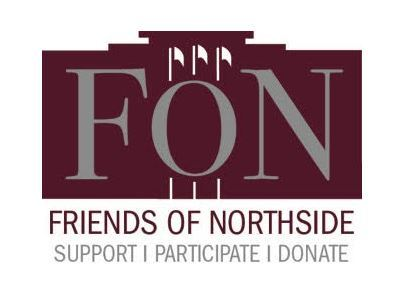# Friends of Northside Meeting

Tuesday, January 22
7:00 pm
Faculty Lounge

 table div table+table+table+table+table+table+table+table+table+table+table+table+table+table+table+table+table+table+table+table+table+table+table+table+table+table+table+table+table+table+table+table+table+table+table+table+table div table{width:100%;padding:0}table div table+table+table+table+table+table+table+table+table+table+table+table+table+table+table+table+table+table+table+table+table+table+table+table+table+table+table+table+table+table+table+table+table+table+table+table+table div table img{width:96.23%;padding:0;float:none}table div table+table+table+table+table+table+table+table+table+table+table+table+table+table+table+table+table+table+table+table+table+table+table+table+table+table+table+table+table+table+table+table+table+table+table+table+table div table td{width:100%;padding:0 1.88% 18px}/* styles */## Purchase Yearbook Personal Ads for Seniors

All materials are due by Friday, March 17.

Commemorate your student's accomplishments or declare your best wishes with a personal ad in the upcoming Stampede. The prices are:

▪ Full Page \$150
▪ Half Page \$80
▪ Quarter Page \$50

Members of the Stampede can create the ad for you, or you can submit a pre-made ad yourself (photo resolution no less than 600 dpi, please). If you choose to submit a pre-made ad, please also email the digital photos as separate attachments.

Questions? Contact Marion McCreedy, Stampede adviser, at mkmccreedy@cps.edu.## And While We're Talking About Yearbooks...

Order yours now to avoid the March 1 price increase!

Yearbooks were not included as an item on the school fee schedule this year.

2) Enter code 4187219

 table div table+table+table+table+table+table+table+table+table+table+table+table+table+table+table+table+table+table+table+table+table+table+table+table+table+table+table+table+table+table+table+table+table+table+table+table+table+table+table+table+table div table{width:100%;padding:0}table div table+table+table+table+table+table+table+table+table+table+table+table+table+table+table+table+table+table+table+table+table+table+table+table+table+table+table+table+table+table+table+table+table+table+table+table+table+table+table+table+table div table img{width:96.23%;padding:0;float:none}table div table+table+table+table+table+table+table+table+table+table+table+table+table+table+table+table+table+table+table+table+table+table+table+table+table+table+table+table+table+table+table+table+table+table+table+table+table+table+table+table+table div table td{width:100%;padding:0 1.88% 18px}/* styles */# Juniors - Class of 2020! Save the Dates!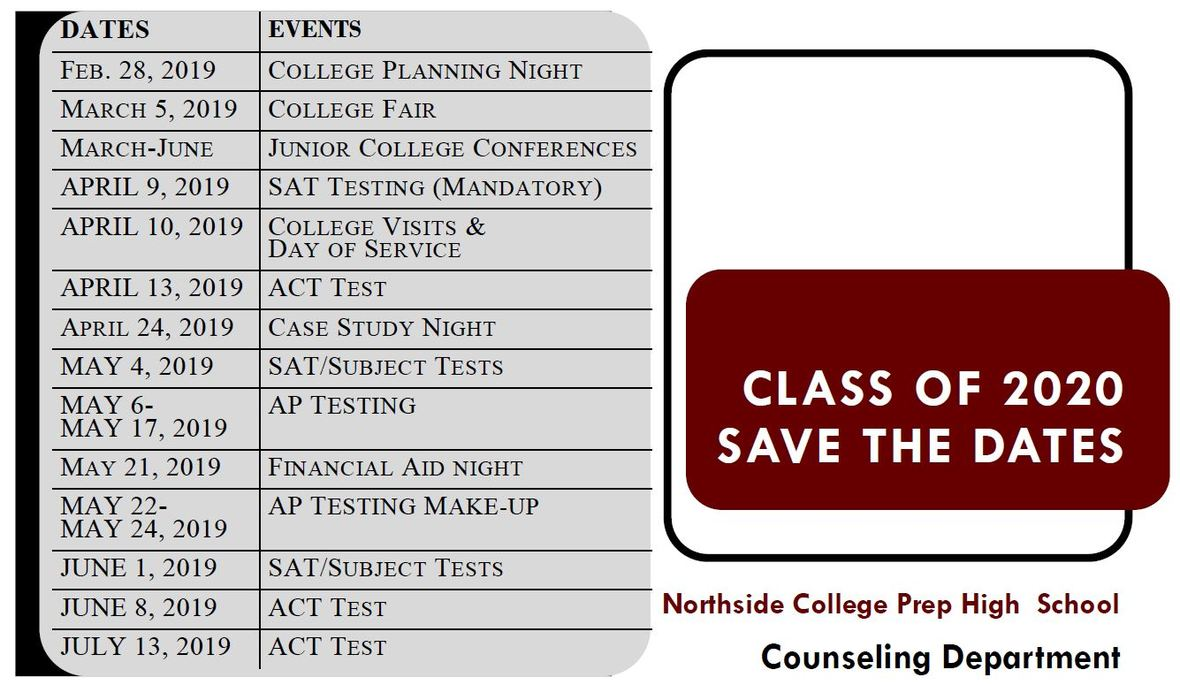table div table+table+table+table+table+table+table+table+table+table+table+table+table+table+table+table+table+table+table+table+table+table+table+table+table+table+table+table+table+table+table+table+table+table+table+table+table+table+table+table+table+table+table+table div table{width:100%;padding:0}table div table+table+table+table+table+table+table+table+table+table+table+table+table+table+table+table+table+table+table+table+table+table+table+table+table+table+table+table+table+table+table+table+table+table+table+table+table+table+table+table+table+table+table+table div table img{width:96.23%;padding:0;float:none}table div table+table+table+table+table+table+table+table+table+table+table+table+table+table+table+table+table+table+table+table+table+table+table+table+table+table+table+table+table+table+table+table+table+table+table+table+table+table+table+table+table+table+table+table div table td{width:100%;padding:0 1.88% 18px}/* styles */Ms. Woodruff is hoping to run a sewing colloquium this spring but needs sewing machines. If you have a sewing machine you can donate, please contact Ms. Woodruff at jwoodruff@cps.edu.

 table div table+table+table+table+table+table+table+table+table+table+table+table+table+table+table+table+table+table+table+table+table+table+table+table+table+table+table+table+table+table+table+table+table+table+table+table+table+table+table+table+table+table+table+table+table+table div table{width:100%;padding:0}table div table+table+table+table+table+table+table+table+table+table+table+table+table+table+table+table+table+table+table+table+table+table+table+table+table+table+table+table+table+table+table+table+table+table+table+table+table+table+table+table+table+table+table+table+table+table div table img{width:96.23%;padding:0;float:none}table div table+table+table+table+table+table+table+table+table+table+table+table+table+table+table+table+table+table+table+table+table+table+table+table+table+table+table+table+table+table+table+table+table+table+table+table+table+table+table+table+table+table+table+table+table+table div table td{width:100%;padding:0 1.88% 18px}/* styles */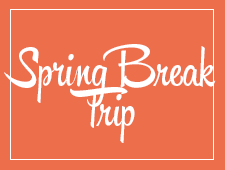# Travel this Spring with Northside

## Spring Break 2019 Trip to Spain

Four amazing cities and more than 15 sites to visit, including Madrid, Granada, Sevilla, and Costa del Sol!

What is included? 8 days of beautiful and historical España! Airfare, all hotel stays, transportation-comfortable coach bus, tour guides, breakfast and dinner, etc.

Open to ALL students.

## Spring Break 2019 Trip to England

On this trip you will experience the distinct character that is London. Admire St Paul's Cathedral, Big Ben,The House of Parliament, and Buckingham Palace. We will also travel to Somerset and visit Stonehenge the United Kingdom's most mysterious landmark, Bath, Stratford (see where William Shakespeare was born), and Oxford.

See Ms. Fernandez or Ms. Vosnos or click HERE for an informational brochure.

## Spring Break 2019 Trip to the Grand Canyon

Students will have a chance to connect with the majesty of our National Park system. We will spend 5 nights in the Grand Canyon camping, hiking, stargazing, and learning wilderness skills. Students will participate in a second-semester colloquium to prepare them for the trip. Trip sponsors and chaperones include Ms. Qazi, Ms. Schaldenbrand, and Mr. Barry Smith. Approximate cost: \$1,000 (includes flight, food, and lodging).

 table div table+table+table+table+table+table+table+table+table+table+table+table+table+table+table+table+table+table+table+table+table+table+table+table+table+table+table+table+table+table+table+table+table+table+table+table+table+table+table+table+table+table+table+table+table+table+table+table div table{width:100%;padding:0}table div table+table+table+table+table+table+table+table+table+table+table+table+table+table+table+table+table+table+table+table+table+table+table+table+table+table+table+table+table+table+table+table+table+table+table+table+table+table+table+table+table+table+table+table+table+table+table+table div table img{width:96.23%;padding:0;float:none}table div table+table+table+table+table+table+table+table+table+table+table+table+table+table+table+table+table+table+table+table+table+table+table+table+table+table+table+table+table+table+table+table+table+table+table+table+table+table+table+table+table+table+table+table+table+table+table+table div table td{width:100%;padding:0 1.88% 18px}/* styles */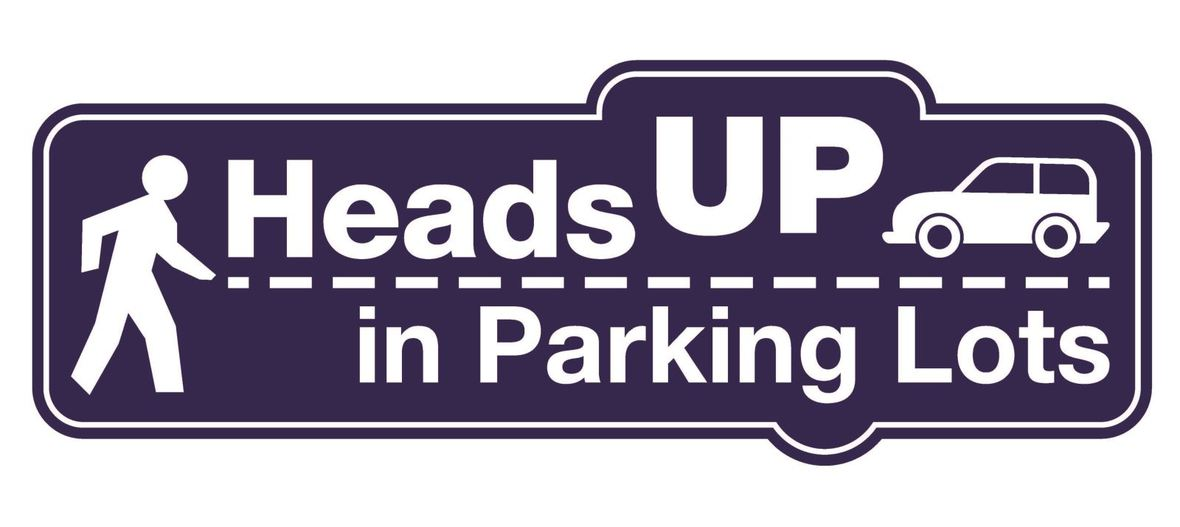# Parking Lot Safety and Procedures

Our NCP school parking lot is a busy place before and after school. Families dropping off and picking up students are asked to be mindful of both safety and courtesy. Please assist us by ensuring that traffic lanes stay clear during drop-off and pick-up times. Pulling over to the curb on the right as your student enters or exits your vehicle keeps students safe and traffic flow efficient.

Please remember that ALL VEHICLES MUST EXIT ONTO KEDZIE. You may enter the parking lot from Kedzie or Bryn Mawr, but regardless of where you enter, all cars must exit onto Kedzie. For the safety of our students and the rest of the Northside community, please do not exit onto Bryn Mawr.

 table div table+table+table+table+table+table+table+table+table+table+table+table+table+table+table+table+table+table+table+table+table+table+table+table+table+table+table+table+table+table+table+table+table+table+table+table+table+table+table+table+table+table+table+table+table+table+table+table+table+table+table div table{width:100%;padding:0}table div table+table+table+table+table+table+table+table+table+table+table+table+table+table+table+table+table+table+table+table+table+table+table+table+table+table+table+table+table+table+table+table+table+table+table+table+table+table+table+table+table+table+table+table+table+table+table+table+table+table+table div table img{width:96.23%;padding:0;float:none}table div table+table+table+table+table+table+table+table+table+table+table+table+table+table+table+table+table+table+table+table+table+table+table+table+table+table+table+table+table+table+table+table+table+table+table+table+table+table+table+table+table+table+table+table+table+table+table+table+table+table+table div table td{width:100%;padding:0 1.88% 18px}/* styles */# CPS Volunteer Requirements

According to CPS policy, anybody who is going to be in contact with students must be approved as a CPS volunteer. There are two levels of volunteers, Level 1 and Level 2.

## Level II Volunteers

(This process can be completed in a day & is required even of volunteers for Friends of Northside events)
A fingerprint criminal background check is not required for Level II Volunteers.

A Level II Volunteer is:

1. A parent providing supervised volunteer service in their child’s school or classroom for less than 10 hours/week;
2. Any individual providing volunteer service for less than 5 hours/week at a school where s/he does not have a child enrolled;
3. An individual providing incidental volunteer service with no ongoing individualized interaction with a student(s) including those who speak at a class/assembly, judge academic competitions, give musical performance, participate in the “Principal for a Day” program, job shadowing event or other one-time event provided where there is direct supervision of the activity/event by regular school employees;
4. A parent accompanying his/her child’s class on a one-day field trip or another type of extracurricular activity that does not involve an overnight stay; and/or
5. An individual providing volunteer service on projects/activities involving no or nominal contact with children (home-based volunteer activities, volunteers serving at Central or Network office).
 1 A parent providing supervised volunteer service in their child’s school or classroom for less than 10 hours/week;
 2 Any individual providing volunteer service for less than 5 hours/week at a school where s/he does not have a child enrolled;
 3 An individual providing incidental volunteer service with no ongoing individualized interaction with a student(s) including those who speak at a class/assembly, judge academic competitions, give musical performance, participate in the “Principal for a Day” program, job shadowing event or other one-time event provided where there is direct supervision of the activity/event by regular school employees;
 4 A parent accompanying his/her child’s class on a one-day field trip or another type of extracurricular activity that does not involve an overnight stay; and/or
 5 An individual providing volunteer service on projects/activities involving no or nominal contact with children (home-based volunteer activities, volunteers serving at Central or Network office).

## Level I Volunteers

(Please allow 8-12 weeks for the entire process)
A fingerprint criminal background check is required for all Level I Volunteers.

A Level I volunteer is:

1. A parent providing volunteer service in their child’s school or classroom for 10 or more hours/week;
2. An individual providing volunteer service for 5 or more hours/week at a school where s/he does not have a child enrolled; and/or
3. Any individual chaperoning an overnight school-sponsored trip, regardless of the hours/week that the volunteer serves (See also the Student Travel Policy).
 1 A parent providing volunteer service in their child’s school or classroom for 10 or more hours/week;
 2 An individual providing volunteer service for 5 or more hours/week at a school where s/he does not have a child enrolled; and/or
 3 Any individual chaperoning an overnight school-sponsored trip, regardless of the hours/week that the volunteer serves (See also the Student Travel Policy).

To become a volunteer, please visit CPS' Volunteer website and follow the instructions. There is also a link on Northside's website on the Parents page Northside Volunteers.

Assistant Principal Kaitlin O'Hare is Northside's Volunteer Coordinator. After applying to become a volunteer, you must visit the school and show Ms. O'Hare your ID, so she can approve you to move forward in the volunteer process. For Level II volunteers, that is the only step you must take. For Level I, you will receive further instructions from CPS.

 table div table+table+table+table+table+table+table+table+table+table+table+table+table+table+table+table+table+table+table+table+table+table+table+table+table+table+table+table+table+table+table+table+table+table+table+table+table+table+table+table+table+table+table+table+table+table+table+table+table+table+table+table+table div table{width:100%;padding:0}table div table+table+table+table+table+table+table+table+table+table+table+table+table+table+table+table+table+table+table+table+table+table+table+table+table+table+table+table+table+table+table+table+table+table+table+table+table+table+table+table+table+table+table+table+table+table+table+table+table+table+table+table+table div table img{width:96.23%;padding:0;float:none}table div table+table+table+table+table+table+table+table+table+table+table+table+table+table+table+table+table+table+table+table+table+table+table+table+table+table+table+table+table+table+table+table+table+table+table+table+table+table+table+table+table+table+table+table+table+table+table+table+table+table+table+table+table div table td{width:100%;padding:0 1.88% 18px}/* styles */table.module-53{width:94.34%;padding:0}table div table+table+table+table+table+table+table+table+table+table+table+table+table+table+table+table+table+table+table+table+table+table+table+table+table+table+table+table+table+table+table+table+table+table+table+table+table+table+table+table+table+table+table+table+table+table+table+table+table+table+table+table+table+table div table{width:94.34%;float:none;margin-left:auto;margin-right:auto;padding:0}table div table+table+table+table+table+table+table+table+table+table+table+table+table+table+table+table+table+table+table+table+table+table+table+table+table+table+table+table+table+table+table+table+table+table+table+table+table+table+table+table+table+table+table+table+table+table+table+table+table+table+table+table+table+table div table a{border:0 none;text-decoration:none}table div table+table+table+table+table+table+table+table+table+table+table+table+table+table+table+table+table+table+table+table+table+table+table+table+table+table+table+table+table+table+table+table+table+table+table+table+table+table+table+table+table+table+table+table+table+table+table+table+table+table+table+table+table+table div table img{width:100%!important;border:0 none;text-decoration:none}table div table+table+table+table+table+table+table+table+table+table+table+table+table+table+table+table+table+table+table+table+table+table+table+table+table+table+table+table+table+table+table+table+table+table+table+table+table+table+table+table+table+table+table+table+table+table+table+table+table+table+table+table+table+table div table td{width:100%;padding:0}/* styles */
 /* styles */ Check out The HoofBeat, Northside's online newspaper, to get NCP news from the student perspective!
 table div table+table+table+table+table+table+table+table+table+table+table+table+table+table+table+table+table+table+table+table+table+table+table+table+table+table+table+table+table+table+table+table+table+table+table+table+table+table+table+table+table+table+table+table+table+table+table+table+table+table+table+table+table+table+table+table div table{width:100%;padding:0}table div table+table+table+table+table+table+table+table+table+table+table+table+table+table+table+table+table+table+table+table+table+table+table+table+table+table+table+table+table+table+table+table+table+table+table+table+table+table+table+table+table+table+table+table+table+table+table+table+table+table+table+table+table+table+table+table div table img{width:96.23%;padding:0;float:none}table div table+table+table+table+table+table+table+table+table+table+table+table+table+table+table+table+table+table+table+table+table+table+table+table+table+table+table+table+table+table+table+table+table+table+table+table+table+table+table+table+table+table+table+table+table+table+table+table+table+table+table+table+table+table+table+table div table td{width:100%;padding:0 1.88% 18px}/* styles */table.module-56{width:57.17%;padding:0}table div table+table+table+table+table+table+table+table+table+table+table+table+table+table+table+table+table+table+table+table+table+table+table+table+table+table+table+table+table+table+table+table+table+table+table+table+table+table+table+table+table+table+table+table+table+table+table+table+table+table+table+table+table+table+table+table+table div table{width:57.17%;float:none;margin-left:auto;margin-right:auto;padding:0}table div table+table+table+table+table+table+table+table+table+table+table+table+table+table+table+table+table+table+table+table+table+table+table+table+table+table+table+table+table+table+table+table+table+table+table+table+table+table+table+table+table+table+table+table+table+table+table+table+table+table+table+table+table+table+table+table+table div table a{border:0 none;text-decoration:none}table div table+table+table+table+table+table+table+table+table+table+table+table+table+table+table+table+table+table+table+table+table+table+table+table+table+table+table+table+table+table+table+table+table+table+table+table+table+table+table+table+table+table+table+table+table+table+table+table+table+table+table+table+table+table+table+table+table div table img{width:100%!important;border:0 none;text-decoration:none}table div table+table+table+table+table+table+table+table+table+table+table+table+table+table+table+table+table+table+table+table+table+table+table+table+table+table+table+table+table+table+table+table+table+table+table+table+table+table+table+table+table+table+table+table+table+table+table+table+table+table+table+table+table+table+table+table+table div table td{width:100%;padding:0}/* styles */

# What is FAN?

FAN is Family Action Network, an organization which connects parents, educators, and professionals through collaborative programming that educates, inspires, and positively impacts the broader community. Nearly every week during the school year there will be a dynamic and important speaker, discussing the issues that interest you.

FAN events are free to all.

# Frederick Douglass: Prophet of Freedom

David Blight, Ph.D.
Class of 1954 Professor of American History and Director of the Gilder Lehrman Center for the Study of Slavery, Resistance, and Abolition, Yale University.
Recipient of the Bancroft Prize, the Abraham Lincoln Prize, and the Frederick Douglass Prize, among others.
Author or editor of a dozen books, including American Oracle: The Civil War in the Civil Rights Era; Race and Reunion: The Civil War in American Memory; and the brand-new Frederick Douglass: Prophet of Freedom
Friday, Jan. 25, 2019
7:00 pm
Evanston Township High School Auditorium
1600 Dodge Ave., Evanston

# Out of Many Faiths: Religious Diversity and the American Promise

Eboo Patel
Founder and President of Interfaith Youth Core
Wednesday, Jan. 30, 2019
7:00 pm
Evanston Township High School Auditorium
1600 Dodge Ave., Evanston

 table div table+table+table+table+table+table+table+table+table+table+table+table+table+table+table+table+table+table+table+table+table+table+table+table+table+table+table+table+table+table+table+table+table+table+table+table+table+table+table+table+table+table+table+table+table+table+table+table+table+table+table+table+table+table+table+table+table+table+table div table{width:100%;padding:0}table div table+table+table+table+table+table+table+table+table+table+table+table+table+table+table+table+table+table+table+table+table+table+table+table+table+table+table+table+table+table+table+table+table+table+table+table+table+table+table+table+table+table+table+table+table+table+table+table+table+table+table+table+table+table+table+table+table+table+table div table img{width:96.23%;padding:0;float:none}table div table+table+table+table+table+table+table+table+table+table+table+table+table+table+table+table+table+table+table+table+table+table+table+table+table+table+table+table+table+table+table+table+table+table+table+table+table+table+table+table+table+table+table+table+table+table+table+table+table+table+table+table+table+table+table+table+table+table+table div table td{width:100%;padding:0 1.88% 18px}/* styles */# Do you have news or team results to share with the Northside parent community?

 table div table+table+table+table+table+table+table+table+table+table+table+table+table+table+table+table+table+table+table+table+table+table+table+table+table+table+table+table+table+table+table+table+table+table+table+table+table+table+table+table+table+table+table+table+table+table+table+table+table+table+table+table+table+table+table+table+table+table+table+table+table+table div table{width:100%;padding:0}table div table+table+table+table+table+table+table+table+table+table+table+table+table+table+table+table+table+table+table+table+table+table+table+table+table+table+table+table+table+table+table+table+table+table+table+table+table+table+table+table+table+table+table+table+table+table+table+table+table+table+table+table+table+table+table+table+table+table+table+table+table+table div table img{width:96.23%;padding:0;float:none}table div table+table+table+table+table+table+table+table+table+table+table+table+table+table+table+table+table+table+table+table+table+table+table+table+table+table+table+table+table+table+table+table+table+table+table+table+table+table+table+table+table+table+table+table+table+table+table+table+table+table+table+table+table+table+table+table+table+table+table+table+table+table div table td{width:100%;padding:0 1.88% 18px}/* styles */# FON Connect

Friends of Northside uses a customer/constituent relationship management (CRM) system known as FON Connect that allows our parent volunteer organizations to work from one database. It is not a CPS database and is completely different and separate from the CPS Parent Portal and Northside's EPay website.

FON Connect serves as the NCP Family Directory and allows users to review their donation history, update their contact information, register for upcoming events, and more. Each parent has a separate account. Transactions, including donations, are recorded in the account of the parent who initiated the transaction or donation.

If you’re new to FON Connect, you'll need to set up a login and password. To do so:

 table div table+table+table+table+table+table+table+table+table+table+table+table+table+table+table+table+table+table+table+table+table+table+table+table+table+table+table+table+table+table+table+table+table+table+table+table+table+table+table+table+table+table+table+table+table+table+table+table+table+table+table+table+table+table+table+table+table+table+table+table+table+table+table+table div table{width:100%;padding:0}table div table+table+table+table+table+table+table+table+table+table+table+table+table+table+table+table+table+table+table+table+table+table+table+table+table+table+table+table+table+table+table+table+table+table+table+table+table+table+table+table+table+table+table+table+table+table+table+table+table+table+table+table+table+table+table+table+table+table+table+table+table+table+table+table div table img{width:96.23%;padding:0;float:none}table div table+table+table+table+table+table+table+table+table+table+table+table+table+table+table+table+table+table+table+table+table+table+table+table+table+table+table+table+table+table+table+table+table+table+table+table+table+table+table+table+table+table+table+table+table+table+table+table+table+table+table+table+table+table+table+table+table+table+table+table+table+table+table+table div table td{width:100%;padding:0 1.88% 18px}/* styles */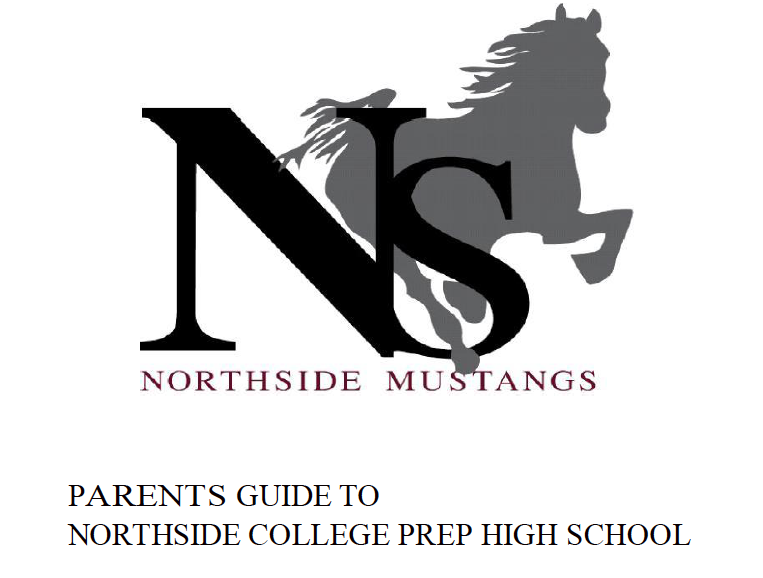# Navigating Northside

Navigating Northside, our parent handbook, is available on the school website under the Parents tab. Click HERE to access it directly.

 table div table+table+table+table+table+table+table+table+table+table+table+table+table+table+table+table+table+table+table+table+table+table+table+table+table+table+table+table+table+table+table+table+table+table+table+table+table+table+table+table+table+table+table+table+table+table+table+table+table+table+table+table+table+table+table+table+table+table+table+table+table+table+table+table+table+table div table{width:100%;padding:0}table div table+table+table+table+table+table+table+table+table+table+table+table+table+table+table+table+table+table+table+table+table+table+table+table+table+table+table+table+table+table+table+table+table+table+table+table+table+table+table+table+table+table+table+table+table+table+table+table+table+table+table+table+table+table+table+table+table+table+table+table+table+table+table+table+table+table div table img{width:96.23%;padding:0;float:none}table div table+table+table+table+table+table+table+table+table+table+table+table+table+table+table+table+table+table+table+table+table+table+table+table+table+table+table+table+table+table+table+table+table+table+table+table+table+table+table+table+table+table+table+table+table+table+table+table+table+table+table+table+table+table+table+table+table+table+table+table+table+table+table+table+table+table div table td{width:100%;padding:0 1.88% 18px}/* styles */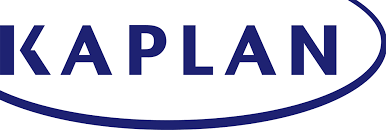# Naviance CCLR Live by Kaplan

## College, Career, and Life Readiness# NCP College Planning Handbook

This is a great resource that guides students through the entire college process. Click HERE to access a copy.

 table div table+table+table+table+table+table+table+table+table+table+table+table+table+table+table+table+table+table+table+table+table+table+table+table+table+table+table+table+table+table+table+table+table+table+table+table+table+table+table+table+table+table+table+table+table+table+table+table+table+table+table+table+table+table+table+table+table+table+table+table+table+table+table+table+table+table+table+table+table+table div table{width:100%;padding:0}table div table+table+table+table+table+table+table+table+table+table+table+table+table+table+table+table+table+table+table+table+table+table+table+table+table+table+table+table+table+table+table+table+table+table+table+table+table+table+table+table+table+table+table+table+table+table+table+table+table+table+table+table+table+table+table+table+table+table+table+table+table+table+table+table+table+table+table+table+table+table div table img{width:96.23%;padding:0;float:none}table div table+table+table+table+table+table+table+table+table+table+table+table+table+table+table+table+table+table+table+table+table+table+table+table+table+table+table+table+table+table+table+table+table+table+table+table+table+table+table+table+table+table+table+table+table+table+table+table+table+table+table+table+table+table+table+table+table+table+table+table+table+table+table+table+table+table+table+table+table+table div table td{width:100%;padding:0 1.88% 18px}/* styles */# Help Northside When You Shop Online

## You can help our school with no extra cost to you.

AmazonSmile is a simple and automatic way to support Northside while you shop.

To choose Northside, please note that our AmazonSmile name is Friends of "North" "Side" (North Side - two words at AmazonSmile).

 table div table+table+table+table+table+table+table+table+table+table+table+table+table+table+table+table+table+table+table+table+table+table+table+table+table+table+table+table+table+table+table+table+table+table+table+table+table+table+table+table+table+table+table+table+table+table+table+table+table+table+table+table+table+table+table+table+table+table+table+table+table+table+table+table+table+table+table+table+table+table+table+table+table div table{width:100%;padding:0}table div table+table+table+table+table+table+table+table+table+table+table+table+table+table+table+table+table+table+table+table+table+table+table+table+table+table+table+table+table+table+table+table+table+table+table+table+table+table+table+table+table+table+table+table+table+table+table+table+table+table+table+table+table+table+table+table+table+table+table+table+table+table+table+table+table+table+table+table+table+table+table+table+table div table img{width:96.23%;padding:0;float:none}table div table+table+table+table+table+table+table+table+table+table+table+table+table+table+table+table+table+table+table+table+table+table+table+table+table+table+table+table+table+table+table+table+table+table+table+table+table+table+table+table+table+table+table+table+table+table+table+table+table+table+table+table+table+table+table+table+table+table+table+table+table+table+table+table+table+table+table+table+table+table+table+table+table div table td{width:100%;padding:0 1.88% 18px}/* styles */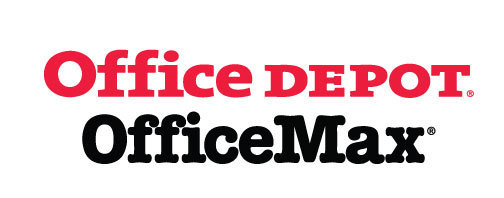# Give Back to Northside

Purchase school and office supplies at Office Depot and OfficeMax, and Northside can receive 5% back in credits or FREE supplies. Simply provide our school ID 70031171 at checkout, online or in stores. There’s no cost to you!

 table div table+table+table+table+table+table+table+table+table+table+table+table+table+table+table+table+table+table+table+table+table+table+table+table+table+table+table+table+table+table+table+table+table+table+table+table+table+table+table+table+table+table+table+table+table+table+table+table+table+table+table+table+table+table+table+table+table+table+table+table+table+table+table+table+table+table+table+table+table+table+table+table+table+table+table+table div table{width:100%;padding:0}table div table+table+table+table+table+table+table+table+table+table+table+table+table+table+table+table+table+table+table+table+table+table+table+table+table+table+table+table+table+table+table+table+table+table+table+table+table+table+table+table+table+table+table+table+table+table+table+table+table+table+table+table+table+table+table+table+table+table+table+table+table+table+table+table+table+table+table+table+table+table+table+table+table+table+table+table div table img{width:96.23%;padding:0;float:none}table div table+table+table+table+table+table+table+table+table+table+table+table+table+table+table+table+table+table+table+table+table+table+table+table+table+table+table+table+table+table+table+table+table+table+table+table+table+table+table+table+table+table+table+table+table+table+table+table+table+table+table+table+table+table+table+table+table+table+table+table+table+table+table+table+table+table+table+table+table+table+table+table+table+table+table+table div table td{width:100%;padding:0 1.88% 18px}/* styles */# Follow Us on Social Media

▪ Northside Prep Parents' Facebook Page, a closed group for parents, by parents, to share their knowledge of Northside
▪ Friends of Northside Facebook Page, for news everyone can view
 ▪ Northside Prep Parents' Facebook Page, a closed group for parents, by parents, to share their knowledge of Northside
 ▪ Friends of Northside Facebook Page, for news everyone can view
 table div table+table+table+table+table+table+table+table+table+table+table+table+table+table+table+table+table+table+table+table+table+table+table+table+table+table+table+table+table+table+table+table+table+table+table+table+table+table+table+table+table+table+table+table+table+table+table+table+table+table+table+table+table+table+table+table+table+table+table+table+table+table+table+table+table+table+table+table+table+table+table+table+table+table+table+table+table+table div table{width:100%;padding:0}table div table+table+table+table+table+table+table+table+table+table+table+table+table+table+table+table+table+table+table+table+table+table+table+table+table+table+table+table+table+table+table+table+table+table+table+table+table+table+table+table+table+table+table+table+table+table+table+table+table+table+table+table+table+table+table+table+table+table+table+table+table+table+table+table+table+table+table+table+table+table+table+table+table+table+table+table+table+table div table img{width:96.23%;padding:0;float:none}table div table+table+table+table+table+table+table+table+table+table+table+table+table+table+table+table+table+table+table+table+table+table+table+table+table+table+table+table+table+table+table+table+table+table+table+table+table+table+table+table+table+table+table+table+table+table+table+table+table+table+table+table+table+table+table+table+table+table+table+table+table+table+table+table+table+table+table+table+table+table+table+table+table+table+table+table+table+table div table td{width:100%;padding:0 1.88% 18px}/* styles */Northside’s website can be found HERE.

Friends of Northside's website can be found HERE.

 table div table+table+table+table+table+table+table+table+table+table+table+table+table+table+table+table+table+table+table+table+table+table+table+table+table+table+table+table+table+table+table+table+table+table+table+table+table+table+table+table+table+table+table+table+table+table+table+table+table+table+table+table+table+table+table+table+table+table+table+table+table+table+table+table+table+table+table+table+table+table+table+table+table+table+table+table+table+table+table+table div table{width:100%;padding:0}table div table+table+table+table+table+table+table+table+table+table+table+table+table+table+table+table+table+table+table+table+table+table+table+table+table+table+table+table+table+table+table+table+table+table+table+table+table+table+table+table+table+table+table+table+table+table+table+table+table+table+table+table+table+table+table+table+table+table+table+table+table+table+table+table+table+table+table+table+table+table+table+table+table+table+table+table+table+table+table+table div table img{width:96.23%;padding:0;float:none}table div table+table+table+table+table+table+table+table+table+table+table+table+table+table+table+table+table+table+table+table+table+table+table+table+table+table+table+table+table+table+table+table+table+table+table+table+table+table+table+table+table+table+table+table+table+table+table+table+table+table+table+table+table+table+table+table+table+table+table+table+table+table+table+table+table+table+table+table+table+table+table+table+table+table+table+table+table+table+table+table div table td{width:100%;padding:0 1.88% 18px}/* styles */